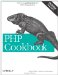# Recipe 2.9. Formatting Numbers

#### 2.9.1. Problem

You have a number and you want to print it with thousands and decimals separators. For example, you want to display the number of people who have viewed a page, or the percentage of people who have voted for an option in a poll.

#### 2.9.2. Solution

Use the number_format( ) function to format as an integer:

`\$number = 1234.56; print number_format(\$number);    // 1,235 because number is rounded up`

Specify a number of decimal places to format as a decimal:

`print number_format(\$number, 2); // 1,234.56`

#### 2.9.3. Discussion

The number_format( ) function formats a number by inserting the correct decimal and thousands separators for your locale. If you want to manually specify these values, pass them as the third and fourth parameters:

`\$number = 1234.56; print number_format(\$number, 2, '@', '#'); // 1#234@56`

The third argument is used as the decimal point and the last separates thousands. If you use these options, you must specify both arguments.

By default, number_format( ) rounds the number to the nearest integer. If you want to preserve the entire number, but you don't know ahead of time how many digits follow the decimal point in your number, use this:

`\$number = 1234.56; // your number list(\$int, \$dec) = explode('.', \$number); print number_format(\$number, strlen(\$dec));`

The localeconv( ) function provides locale-specific data, including number format information. For example:

` setlocale(LC_ALL, 'zh_CN'); print_r(localeconv());  Array (     [decimal_point] => .     [thousands_sep] => ,     [int_curr_symbol] => CNY     [currency_symbol] => ¥     [mon_decimal_point] => .     [mon_thousands_sep] => ,     [positive_sign] =>     [negative_sign] => -     [int_frac_digits] => 0     [frac_digits] => 0     [p_cs_precedes] => 1     [p_sep_by_space] => 0     [n_cs_precedes] => 1     [n_sep_by_space] => 0     [p_sign_posn] => 1     [n_sign_posn] => 4     [grouping] => Array         (              => 3              => 3         )     [mon_grouping] => Array         (              => 3              => 3         ) )  `

Use the decimal_point, thousands_sep, and other settings to see how to format a number for that locale.

Chapter 19 for information on internationalization and localization; documentation on localeconv( ) at http://www.php.net/localeconv and number_format( ) at http://www.php.net/number-format.PHP Cookbook: Solutions and Examples for PHP Programmers
ISBN: 0596101015
EAN: 2147483647
Year: 2006
Pages: 445

Similar book on Amazon